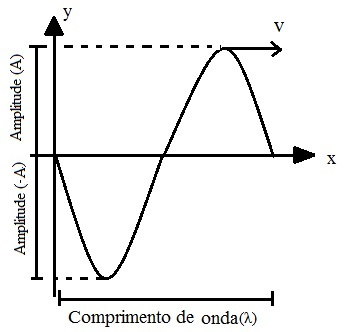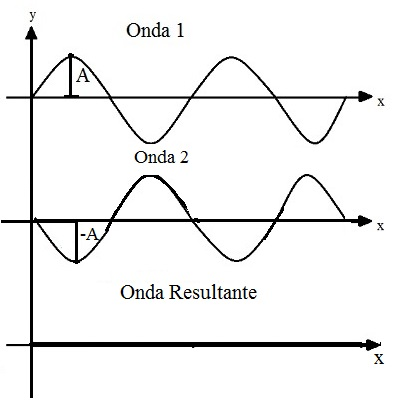Sound and Oscillation

# wave interference

Wave interference is the superposition of two waves in space. This phenomenon can be classified in two ways: constructive or destructive interference.

You must have let your cell phone ring near a computer speakers and noticed that they started to squeak. You must also have heard that, when traveling by plane , the cell phone must be turned off to avoid failures in communication devices. Both cases have the same reason: wave interference .

Waves are pulses of energy that propagate through space periodically. When two waves overlap in the same region of space, interference occurs, which results in another wave with different intensity. These variations in the intensity of the resulting wave are called interference fringes.

This phenomenon was described by Thomas Young in 1801, when he experimentally proved that light is a wave , demonstrating that it is interfered with just like ocean waves.

To understand how interference occurs, we must first know the amplitude and length of a wave . Look the following picture:Amplitude and length of a wave

Amplitude is symbolized by the letter A and represents the height of the wave from the equilibrium point to the maximum height, which is the maximum point of the wave. The negative amplitude represents the distance from the equilibrium point to the minimum point.

The wavelength , represented by the letter λ (lambda), corresponds to the distance between two equal and successive points of a wave pulse. In the following figure, we can see that the wave pulse starts at y = 0 and ends also at y = 0. The change in the x axis between these two intervals is the wavelength.

Interference can be classified into two types:

• Constructive interference: Occurs when the two superimposed waves have the same phase and one “reinforces” the other, resulting in a larger wave than the one that gave rise to it. See the figure:In constructive interference, one wave “reinforces” the other, which results in a wave larger than the original wave.

We can see in this figure that the resulting wave from the combination of the two other waves has the resulting amplitude given by the sum of the individual amplitudes. Mathematically:

The resultant = A 1 + A 2

Being:

1 – wave 1 amplitude
2 – wave 2 amplitude

Since the two waves are equal and A 1 = A 2 = A, we have:

resultant = A + A
resultant = 2A

After the encounter, the two waves propagate again with their initial characteristics.

• Destructive interference: Occurs when two waves that meet have different phases, so that one annihilates the other. See the figure:When the two waves are not in equal phases, interference is destructive and one annihilates the other.

The figure shows that two waves that propagate in different phases, when they meet, cancel each other out. The result is a wave with zero amplitude. Here’s how the calculations are done in this case:

The resultant = A 1 + A 2

Now we have that A 1 = A and A 2 = – A, substituting in the above equation:

resultant = A 1 + A 2
resultant = A + (-A)
resultant = A – A
resultant = 0

In the examples above, we used two waves propagating with equal wavelengths and amplitudes to facilitate the understanding of the content. However, the phenomenon of wave interference also occurs with waves that have different amplitudes, but have the same properties. In this case, the same equations are valid.